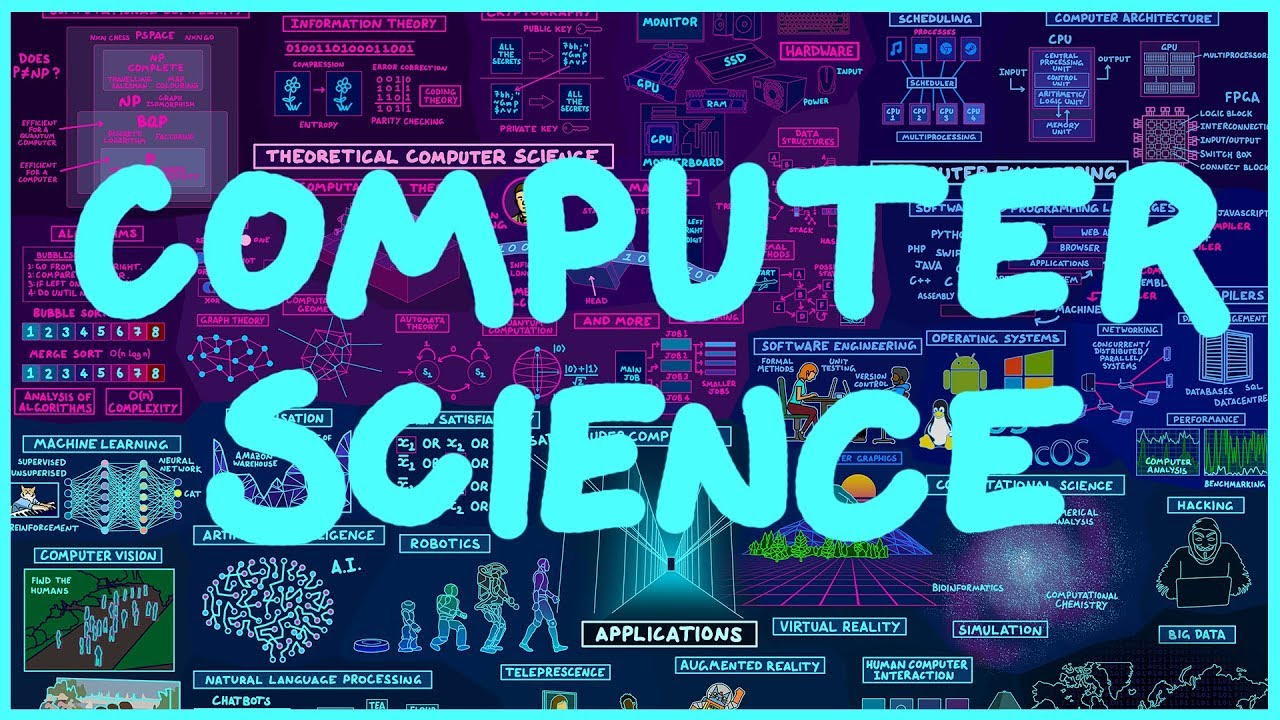## Math’s relation to Computer Science

My career is in computer science . Mathematics relates to my career because every where you turn it deals with math whether it is discrete mathematics or logic. This mainly goes for the understanding of data structures, probability, and anything else when it comes to calculus. If your career is software engineering or information systems, math plays a major role. It will help us gain a deeper understanding in mathematical foundations of their subject and mathematics of application areas, where computers can solve intractable problems. Mathematics used in computer science helps with methods for computer and information sciences, designing , implementation, and analysis of algorithms and software tools for mathematical computation and reasoning.